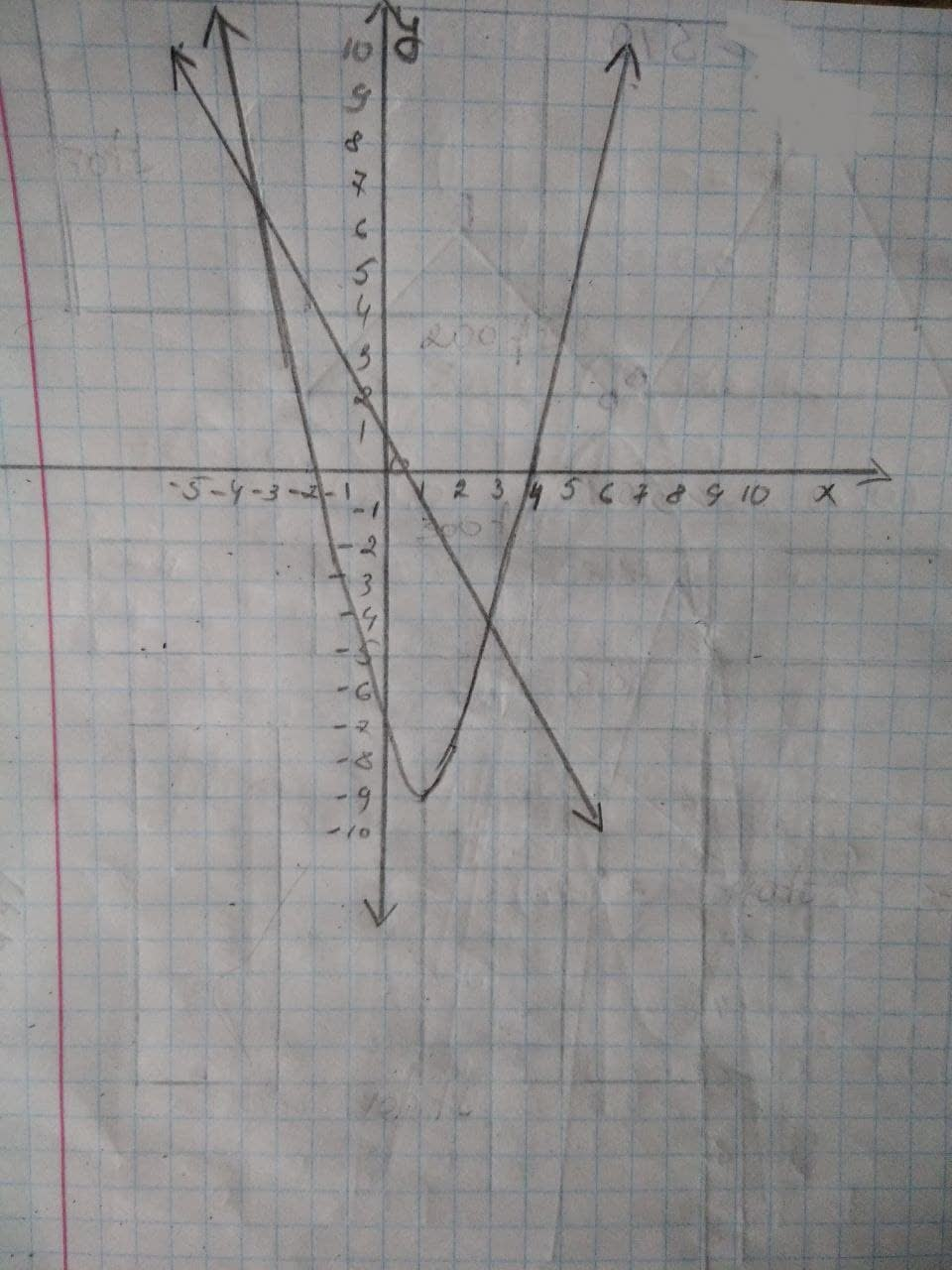# A quadratic-linear system of equations is graphed below. FSP196109Jessie Lee 2021-12-09 Answered
A quadratic-linear system of equations is graphed below.a) Use the graph to write the solution to the system. No need to show any work.
b) Write the equation of the linear function drawn on the grid. No need to show any work.
c) Write the equation of the Quadratic function drawn on the grid in standard form. [Hint: use the x-intercepts]
You can still ask an expert for help

• Live experts 24/7
• Questions are typically answered in as fast as 30 minutes
• Personalized clear answers

Solve your problem for the price of one coffee

• Math expert for every subject
• Pay only if we can solve itjgardner33v4

Step1
Part (a):
Use the graph to write the solution to the system.
There are one quadratic equation and one linear equation.
The intersecting point of both of the graph is the solution of the function.
$\left(-3,7\right)\left(3,5\right)$
Step 2
Part (b):
The equation of the linear function is passing through the point (-3, 7) and (3, -5):
line
slope: $m=\frac{-5-7}{3-\left(-3\right)}=-2$
$y=\left(-2\right)x+b$
Plug in (-3,7) in the equation $y=\left(-2\right)x+b$:
$7=\left(-2\right)\left(-3\right)+b⇒b=1$
$y=-2x+1$
Step 3
The equation of quadratic function $a{x}^{2}+bx=0$ on the grid is passing through (-2, 0), (4, 0) and (0, -8):
$y\left(x\right)=a{x}^{2}+bx+c$
passing through (-2,0),(4,0) and (0,-8):
$0=a{\left(-2\right)}^{2}+b\left(-2\right)+c⇒4a-2b+c=0$
$0=a\left(4\right)+b{\left(4\right)}^{2}+c⇒16a+4b+c=0$
$-8=a\left(0\right)+b\left(0\right)+c⇒c=-8$
$\left[\begin{array}{c}4a-2b-8=0\\ 16a+4b-8=0\end{array}\right]⇒b=-2,a=1$
$a=1,b=-2,c=-8$
$y\left(x\right)={x}^{2}-2x-8$